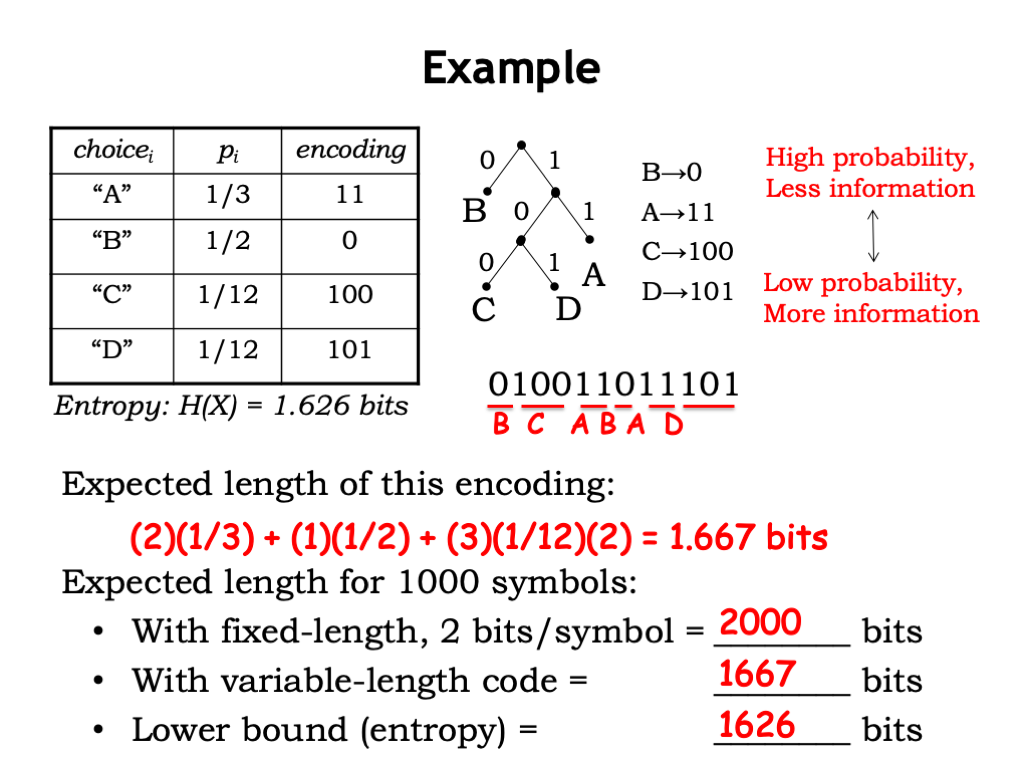# Unsupervised Learning: Information Theory Recap

This is the 13th in a series of class notes as I go through the Georgia Tech/Udacity Machine Learning course. The class textbook is Machine Learning by Tom Mitchell.

⚠️This is a more theoretical chapter, feel free to skip if not directly interested.

## The Central Question🔗

In a machine learning problem, we want to be able to ask questions like "how is X1 or X2 related to Y", or "are X1 and X2 related" (mutual information). In general these machine learning problems can be modeled as a probability density function. So information theory is a mathematical framework which allows us to compare these density functions.

## Information Theory History🔗

Claude Shannon. End of story 😅

## Entropy and the Measurement of information🔗

We measure information in terms of the minimum number of bits we need to encode messages. 10 Coin Flips = 10 bits.Another way of looking at amount of information is the number of granular questions you have to ask in order to determine what the message is. The number of bits per symbol is also know as "entropy".

## Variable Length Encoding🔗

However encoding doesnt have to be strictly based on uniform distribution and even trees - we can change our encoding around based on the probability of more frequent bits of information to save on the average bits per message (aka expected size).## Joint and Conditional Entropy🔗

Some variables have covariance with others. For example, the probability that it is raining (X) and the probability that there will be thunder (Y). This can also be expressed as Joint vs Conditional Entropy:If X and Y are independent, then the conditional entropy is just the same as the individual entropies, and the joint entropy is the SUM of the individual entropies.

## Mutual Information🔗

Although Joint and Conditional entropy give us some insight of independence, they aren't direct measures of dependence. For example, if `H(Y|X)` is small, we don't know if that's because `X` gives a lot of information about `Y`, or if `H(Y)` is just small to begin with.

A better measure normalizes this:

``````I(X,Y) = H(Y) - H(X|Y)
``````

and we call it Mutual information.

## Example🔗

Given this system of equations you can verify that, for 2 independent coins:

• Individual entropy is 1
• Conditional entropy is 1
• Joint entropy is 2 (additive)
• Mutual Information is 0 (no shared information)

Which are intuitive. Vice versa for the fully dependent case.

## Kullback-Leibler (KL) Divergence🔗

Mutual Information is just a special case of KL Divergence - it is a measure of distance or divergence between any two distributions.Note it is always non-negative and equals zero only when `p = q` for all x.

In Machine Learning we can use KL Divergence as an alternative to least-squares fitting of our dataset. (to be explained in later chaptesr)

## Next in our series🔗

Further notes on this topic:

Hopefully that was a good introduction to Information Theory. I am planning more primers and would love your feedback and questions on:

• Overview
• Supervised Learning
• Unsupervised Learning
• Reinforcement Learning
• Markov Decision Processes - week of Mar 25
• "True" RL - week of Apr 1
• Game Theory - week of Apr 15